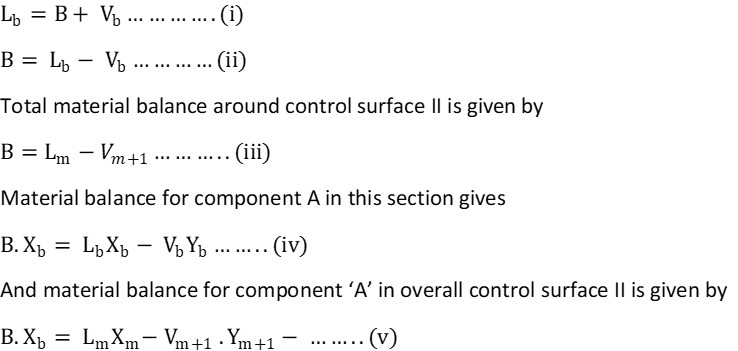# Material Balance for continuous fractionating column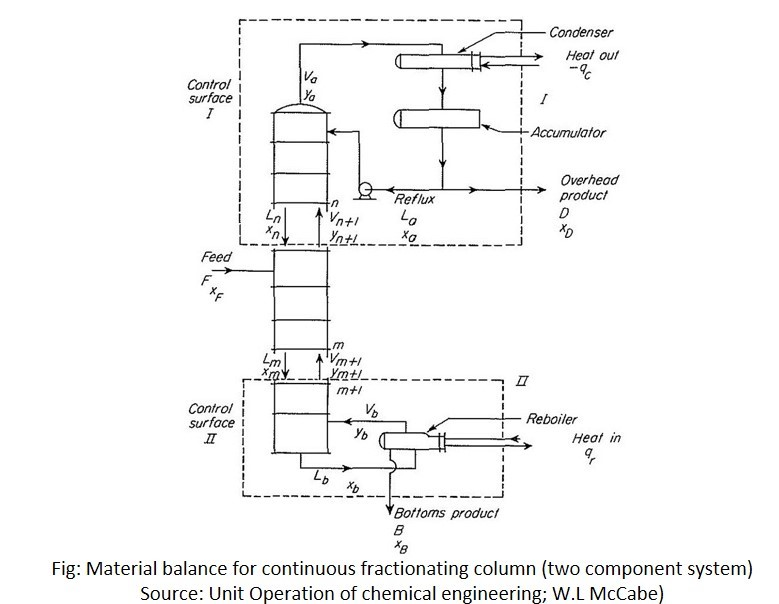In above figure, the column is fed with F mol/h of concentration Xf and delivers D mol/h of overhead product of concentration XD and B mol/h of bottom product of concentration XB.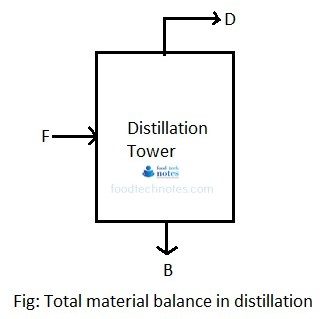The material balance for the system is

F = D +B……………….. (i)

For distillation of two component system, let us consider component A is most volatile component (MVC). Then material balance for most volatile component is given by

F . Xf = D . XD + B . XB………… (ii)

Eliminating B from equation ii gives

F . Xf = D . XD + (F-D) XB

F . Xf = D . XD + F. XB  – D . XB

F . Xf – F. XB = D . XD  – D . XB

F(Xf – XB) = D (XD  – XB)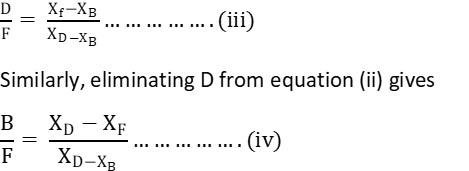Net flow rate:

Difference between vapor and liquid anywhere in the column is given by net flow rate.

Control surface I: This section includes condenser and all plates above n+1. Material balance around condenser and accumulator is given by.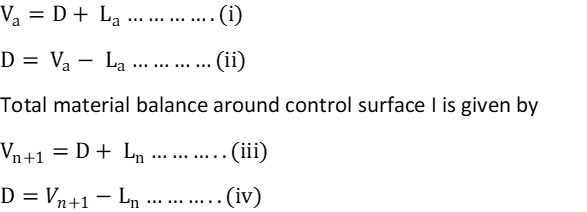Thus net flow rate in this section (control surface I) is given by ‘D’. Regardless of changes in V and L, their difference is constant and equal to ‘D’. Material balance for component A around condenser and accumulator gives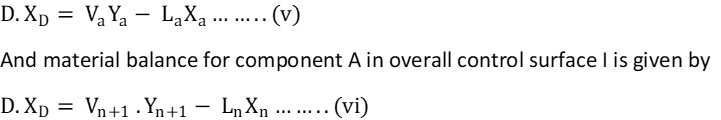Here, quantity  net flow of component ‘A’ upward in upper section of the column, which is constant throughout control surface I.

Control surface II: This is the lower section of column and includes reboiler and all plates below ‘m’. Here, material balance around reboiler is given by